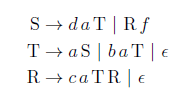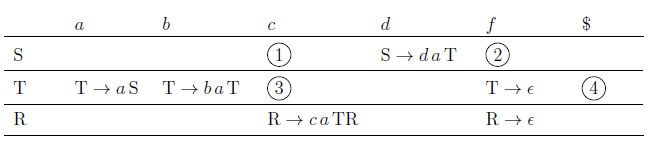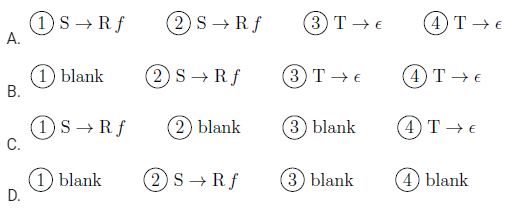Related Articles
GATE | GATE CS 2021 | Set 1 | Question 41
• Difficulty Level : Easy
• Last Updated : 22 Mar, 2021

Consider the following context-free grammar where the set of terminals is {a,b,c,d,f}.The following is a partially-filled LL(1) parsing table.Which one of the following choices represents the correct combination for the numbered cells in the parsing table (“blank” denotes that the corresponding cell is empty)?(A) A
(B) B
(C) C
(D) D

Answer: (A)

Explanation:

Let’s Compute First and Follow of non terminals.

Now using first and follow we will construct LL(1) parsing table.

1. S ->Rf production entry  will come under first of S terminals (c,f) in parsing table.

2. T->∈ production entry  will come under follow of T terminals (c,f,\$) in parsing table.My Personal Notes arrow_drop_up